# Chapter 5 Basic Regression

Now that we are equipped with data visualization skills from Chapter 2, data wrangling skills from Chapter 3, and an understanding of how to import data and the concept of a “tidy” data format from Chapter 4, let’s now proceed with data modeling. The fundamental premise of data modeling is to make explicit the relationship between:

• an outcome variable $$y$$, also called a dependent variable or response variable, and
• an explanatory/predictor variable $$x$$, also called an independent variable or covariate.

Another way to state this is using mathematical terminology: we will model the outcome variable $$y$$ “as a function” of the explanatory/predictor variable $$x$$. When we say “function” here, we aren’t referring to functions in R like the ggplot() function, but rather as a mathematical function. But, why do we have two different labels, explanatory and predictor, for the variable $$x$$? That’s because even though the two terms are often used interchangeably, roughly speaking data modeling serves one of two purposes:

1. Modeling for explanation: When you want to explicitly describe and quantify the relationship between the outcome variable $$y$$ and a set of explanatory variables $$x$$, determine the significance of any relationships, have measures summarizing these relationships, and possibly identify any causal relationships between the variables.
2. Modeling for prediction: When you want to predict an outcome variable $$y$$ based on the information contained in a set of predictor variables $$x$$. Unlike modeling for explanation, however, you don’t care so much about understanding how all the variables relate and interact with one another, but rather only whether you can make good predictions about $$y$$ using the information in $$x$$.

For example, say you are interested in an outcome variable $$y$$ of whether patients develop lung cancer and information $$x$$ on their risk factors, such as smoking habits, age, and socioeconomic status. If we are modeling for explanation, we would be interested in both describing and quantifying the effects of the different risk factors. One reason could be that you want to design an intervention to reduce lung cancer incidence in a population, such as targeting smokers of a specific age group with advertising for smoking cessation programs. If we are modeling for prediction, however, we wouldn’t care so much about understanding how all the individual risk factors contribute to lung cancer, but rather only whether we can make good predictions of which people will contract lung cancer.

In this book, we’ll focus on modeling for explanation and hence refer to $$x$$ as explanatory variables. If you are interested in learning about modeling for prediction, we suggest you check out books and courses on the field of machine learning such as An Introduction to Statistical Learning with Applications in R (ISLR) . Furthermore, while there exist many techniques for modeling, such as tree-based models and neural networks, in this book we’ll focus on one particular technique: linear regression. Linear regression is one of the most commonly-used and easy-to-understand approaches to modeling.

Linear regression involves a numerical outcome variable $$y$$ and explanatory variables $$x$$ that are either numerical or categorical. Furthermore, the relationship between $$y$$ and $$x$$ is assumed to be linear, or in other words, a line. However, we’ll see that what constitutes a “line” will vary depending on the nature of your explanatory variables $$x$$.

In Chapter 5 on basic regression, we’ll only consider models with a single explanatory variable $$x$$. In Section 5.1, the explanatory variable will be numerical. This scenario is known as simple linear regression. In Section 5.2, the explanatory variable will be categorical.

In Chapter 6 on multiple regression, we’ll extend the ideas behind basic regression and consider models with two explanatory variables $$x_1$$ and $$x_2$$. In Section 6.1, we’ll have two numerical explanatory variables. In Section 6.2, we’ll have one numerical and one categorical explanatory variable. In particular, we’ll consider two such models: interaction and parallel slopes models.

In Chapter 10 on inference for regression, we’ll revisit our regression models and analyze the results using the tools for statistical inference you’ll develop in Chapters 7, 8, and 9 on sampling, bootstrapping and confidence intervals, and hypothesis testing and $$p$$-values, respectively.

Let’s now begin with basic regression, which refers to linear regression models with a single explanatory variable $$x$$. We’ll also discuss important statistical concepts like the correlation coefficient, that “correlation isn’t necessarily causation,” and what it means for a line to be “best-fitting.”

### Needed packages

Let’s now load all the packages needed for this chapter (this assumes you’ve already installed them). In this chapter, we introduce some new packages:

1. The tidyverse “umbrella” package. Recall from our discussion in Section 4.4 that loading the tidyverse package by running library(tidyverse) loads the following commonly used data science packages all at once:
• ggplot2 for data visualization
• dplyr for data wrangling
• tidyr for converting data to “tidy” format
• readr for importing spreadsheet data into R
• As well as the more advanced purrr, tibble, stringr, and forcats packages
2. The moderndive package of datasets and functions for tidyverse-friendly introductory linear regression.
3. The skimr package, which provides a simple-to-use function to quickly compute a wide array of commonly used summary statistics.

If needed, read Section 1.3 for information on how to install and load R packages.

library(tidyverse)
library(moderndive)
library(skimr)
library(gapminder)

## 5.1 One numerical explanatory variable

Why do some professors and instructors at universities and colleges receive high teaching evaluations scores from students while others receive lower ones? Are there differences in teaching evaluations between instructors of different demographic groups? Could there be an impact due to student biases? These are all questions that are of interest to university/college administrators, as teaching evaluations are among the many criteria considered in determining which instructors and professors get promoted.

Researchers at the University of Texas in Austin, Texas (UT Austin) tried to answer the following research question: what factors explain differences in instructor teaching evaluation scores? To this end, they collected instructor and course information on 463 courses. A full description of the study can be found at openintro.org.

In this section, we’ll keep things simple for now and try to explain differences in instructor teaching scores as a function of one numerical variable: the instructor’s “beauty” score (we’ll describe how this score was determined shortly). Could it be that instructors with higher “beauty” scores also have higher teaching evaluations? Could it be instead that instructors with higher “beauty” scores tend to have lower teaching evaluations? Or could it be that there is no relationship between “beauty” score and teaching evaluations? We’ll answer these questions by modeling the relationship between teaching scores and “beauty” scores using simple linear regression where we have:

1. A numerical outcome variable $$y$$ (the instructor’s teaching score) and
2. A single numerical explanatory variable $$x$$ (the instructor’s “beauty” score).

### 5.1.1 Exploratory data analysis

The data on the 463 courses at UT Austin can be found in the evals data frame included in the moderndive package. However, to keep things simple, let’s select() only the subset of the variables we’ll consider in this chapter, and save this data in a new data frame called evals_ch5:

evals_ch5 <- evals %>%
select(ID, score, bty_avg, age)

A crucial step before doing any kind of analysis or modeling is performing an exploratory data analysis, or EDA for short. EDA gives you a sense of the distributions of the individual variables in your data, whether any potential relationships exist between variables, whether there are outliers and/or missing values, and (most importantly) how to build your model. Here are three common steps in an EDA:

1. Most crucially, looking at the raw data values.
2. Computing summary statistics, such as means, medians, and interquartile ranges.
3. Creating data visualizations.

Let’s perform the first common step in an exploratory data analysis: looking at the raw data values. Because this step seems so trivial, unfortunately many data analysts ignore it. However, getting an early sense of what your raw data looks like can often prevent many larger issues down the road.

You can do this by using RStudio’s spreadsheet viewer or by using the glimpse() function as introduced in Subsection 1.4.3 on exploring data frames:

glimpse(evals_ch5)
Rows: 463
Columns: 4
$ID <int> 1, 2, 3, 4, 5, 6, 7, 8, 9, 10, 11, 12, 13, 14, 15, 16, 17, 18,…$ score   <dbl> 4.7, 4.1, 3.9, 4.8, 4.6, 4.3, 2.8, 4.1, 3.4, 4.5, 3.8, 4.5, 4.…
$bty_avg <dbl> 5.00, 5.00, 5.00, 5.00, 3.00, 3.00, 3.00, 3.33, 3.33, 3.17, 3.…$ age     <int> 36, 36, 36, 36, 59, 59, 59, 51, 51, 40, 40, 40, 40, 40, 40, 40…

Observe that Rows: 463 indicates that there are 463 rows/observations in evals_ch5, where each row corresponds to one observed course at UT Austin. It is important to note that the observational unit is an individual course and not an individual instructor. Recall from Subsection 1.4.3 that the observational unit is the “type of thing” that is being measured by our variables. Since instructors teach more than one course in an academic year, the same instructor will appear more than once in the data. Hence there are fewer than 463 unique instructors being represented in evals_ch5. We’ll revisit this idea in Section 10.3, when we talk about the “independence assumption” for inference for regression.

A full description of all the variables included in evals can be found at openintro.org or by reading the associated help file (run ?evals in the console). However, let’s fully describe only the 4 variables we selected in evals_ch5:

1. ID: An identification variable used to distinguish between the 1 through 463 courses in the dataset.
2. score: A numerical variable of the course instructor’s average teaching score, where the average is computed from the evaluation scores from all students in that course. Teaching scores of 1 are lowest and 5 are highest. This is the outcome variable $$y$$ of interest.
3. bty_avg: A numerical variable of the course instructor’s average “beauty” score, where the average is computed from a separate panel of six students. “Beauty” scores of 1 are lowest and 10 are highest. This is the explanatory variable $$x$$ of interest.
4. age: A numerical variable of the course instructor’s age. This will be another explanatory variable $$x$$ that we’ll use in the Learning check at the end of this subsection.

An alternative way to look at the raw data values is by choosing a random sample of the rows in evals_ch5 by piping it into the sample_n() function from the dplyr package. Here we set the size argument to be 5, indicating that we want a random sample of 5 rows. We display the results in Table 5.1. Note that due to the random nature of the sampling, you will likely end up with a different subset of 5 rows.

evals_ch5 %>%
sample_n(size = 5)
TABLE 5.1: A random sample of 5 out of the 463 courses at UT Austin
ID score bty_avg age
129 3.7 3.00 62
109 4.7 4.33 46
28 4.8 5.50 62
434 2.8 2.00 62
330 4.0 2.33 64

Now that we’ve looked at the raw values in our evals_ch5 data frame and got a preliminary sense of the data, let’s move on to the next common step in an exploratory data analysis: computing summary statistics. Let’s start by computing the mean and median of our numerical outcome variable score and our numerical explanatory variable “beauty” score denoted as bty_avg. We’ll do this by using the summarize() function from dplyr along with the mean() and median() summary functions we saw in Section 3.3.

evals_ch5 %>%
summarize(mean_bty_avg = mean(bty_avg), mean_score = mean(score),
median_bty_avg = median(bty_avg), median_score = median(score))
# A tibble: 1 × 4
mean_bty_avg mean_score median_bty_avg median_score
<dbl>      <dbl>          <dbl>        <dbl>
1         4.42       4.17           4.33          4.3

However, what if we want other summary statistics as well, such as the standard deviation (a measure of spread), the minimum and maximum values, and various percentiles?

Typing out all these summary statistic functions in summarize() would be long and tedious. Instead, let’s use the convenient skim() function from the skimr package. This function takes in a data frame, “skims” it, and returns commonly used summary statistics. Let’s take our evals_ch5 data frame, select() only the outcome and explanatory variables teaching score and bty_avg, and pipe them into the skim() function:

evals_ch5 %>% select(score, bty_avg) %>% skim()
Skim summary statistics
n obs: 463
n variables: 2

── Variable type:numeric
variable missing complete   n mean   sd   p0  p25  p50 p75 p100
bty_avg       0      463 463 4.42 1.53 1.67 3.17 4.33 5.5 8.17
score       0      463 463 4.17 0.54 2.3  3.8  4.3  4.6 5   

(For formatting purposes in this book, the inline histogram that is usually printed with skim() has been removed. This can be done by using skim_with(numeric = list(hist = NULL)) prior to using the skim() function for version 1.0.6 of skimr.)

For the numerical variables teaching score and bty_avg it returns:

• missing: the number of missing values
• complete: the number of non-missing or complete values
• n: the total number of values
• mean: the average
• sd: the standard deviation
• p0: the 0th percentile: the value at which 0% of observations are smaller than it (the minimum value)
• p25: the 25th percentile: the value at which 25% of observations are smaller than it (the 1st quartile)
• p50: the 50th percentile: the value at which 50% of observations are smaller than it (the 2nd quartile and more commonly called the median)
• p75: the 75th percentile: the value at which 75% of observations are smaller than it (the 3rd quartile)
• p100: the 100th percentile: the value at which 100% of observations are smaller than it (the maximum value)

Looking at this output, we can see how the values of both variables distribute. For example, the mean teaching score was 4.17 out of 5, whereas the mean “beauty” score was 4.42 out of 10. Furthermore, the middle 50% of teaching scores was between 3.80 and 4.6 (the first and third quartiles), whereas the middle 50% of “beauty” scores falls within 3.17 to 5.5 out of 10.

The skim() function only returns what are known as univariate summary statistics: functions that take a single variable and return some numerical summary of that variable. However, there also exist bivariate summary statistics: functions that take in two variables and return some summary of those two variables. In particular, when the two variables are numerical, we can compute the correlation coefficient. Generally speaking, coefficients are quantitative expressions of a specific phenomenon. A correlation coefficient is a quantitative expression of the strength of the linear relationship between two numerical variables. Its value ranges between -1 and 1 where:

• -1 indicates a perfect negative relationship: As one variable increases, the value of the other variable tends to go down, following a straight line.
• 0 indicates no relationship: The values of both variables go up/down independently of each other.
• +1 indicates a perfect positive relationship: As the value of one variable goes up, the value of the other variable tends to go up as well in a linear fashion.

Figure 5.1 gives examples of 9 different correlation coefficient values for hypothetical numerical variables $$x$$ and $$y$$. For example, observe in the top right plot that for a correlation coefficient of -0.75 there is a negative linear relationship between $$x$$ and $$y$$, but it is not as strong as the negative linear relationship between $$x$$ and $$y$$ when the correlation coefficient is -0.9 or -1.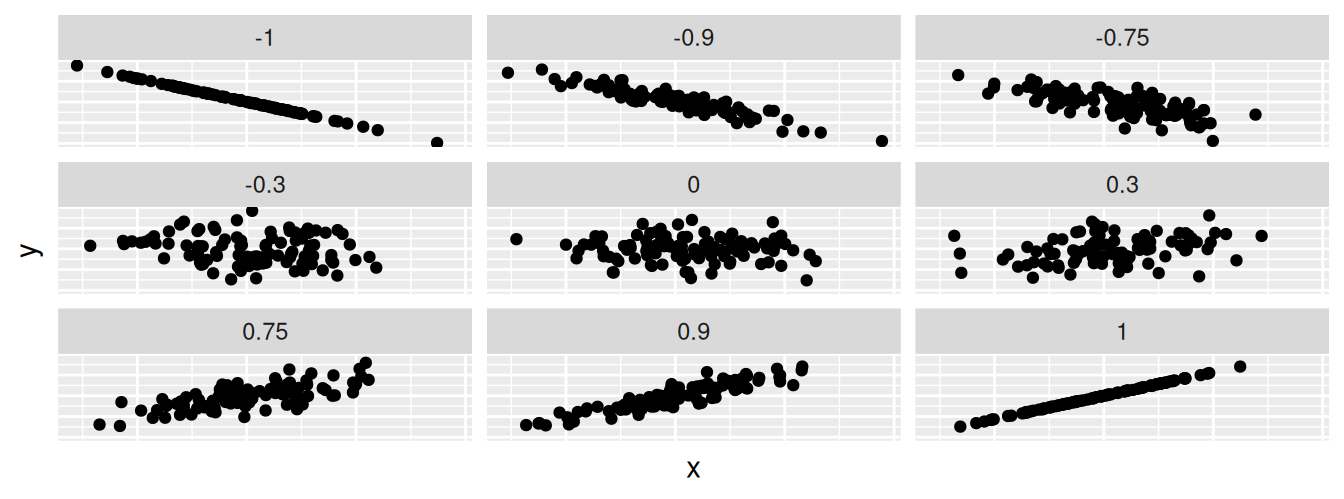FIGURE 5.1: Nine different correlation coefficients.

The correlation coefficient can be computed using the get_correlation() function in the moderndive package. In this case, the inputs to the function are the two numerical variables for which we want to calculate the correlation coefficient.

We put the name of the outcome variable on the left-hand side of the ~ “tilde” sign, while putting the name of the explanatory variable on the right-hand side. This is known as R’s formula notation. We will use this same “formula” syntax with regression later in this chapter.

evals_ch5 %>%
get_correlation(formula = score ~ bty_avg)
# A tibble: 1 × 1
cor
<dbl>
1 0.187

An alternative way to compute correlation is to use the cor() summary function within a summarize():

evals_ch5 %>%
summarize(correlation = cor(score, bty_avg))

In our case, the correlation coefficient of 0.187 indicates that the relationship between teaching evaluation score and “beauty” average is “weakly positive.” There is a certain amount of subjectivity in interpreting correlation coefficients, especially those that aren’t close to the extreme values of -1, 0, and 1. To develop your intuition about correlation coefficients, play the “Guess the Correlation” 1980’s style video game mentioned in Subsection 5.4.1.

Let’s now perform the last of the steps in an exploratory data analysis: creating data visualizations. Since both the score and bty_avg variables are numerical, a scatterplot is an appropriate graph to visualize this data. Let’s do this using geom_point() and display the result in Figure 5.2. Furthermore, let’s highlight the six points in the top right of the visualization in a box.

ggplot(evals_ch5, aes(x = bty_avg, y = score)) +
geom_point() +
labs(x = "Beauty Score",
y = "Teaching Score",
title = "Scatterplot of relationship of teaching and beauty scores")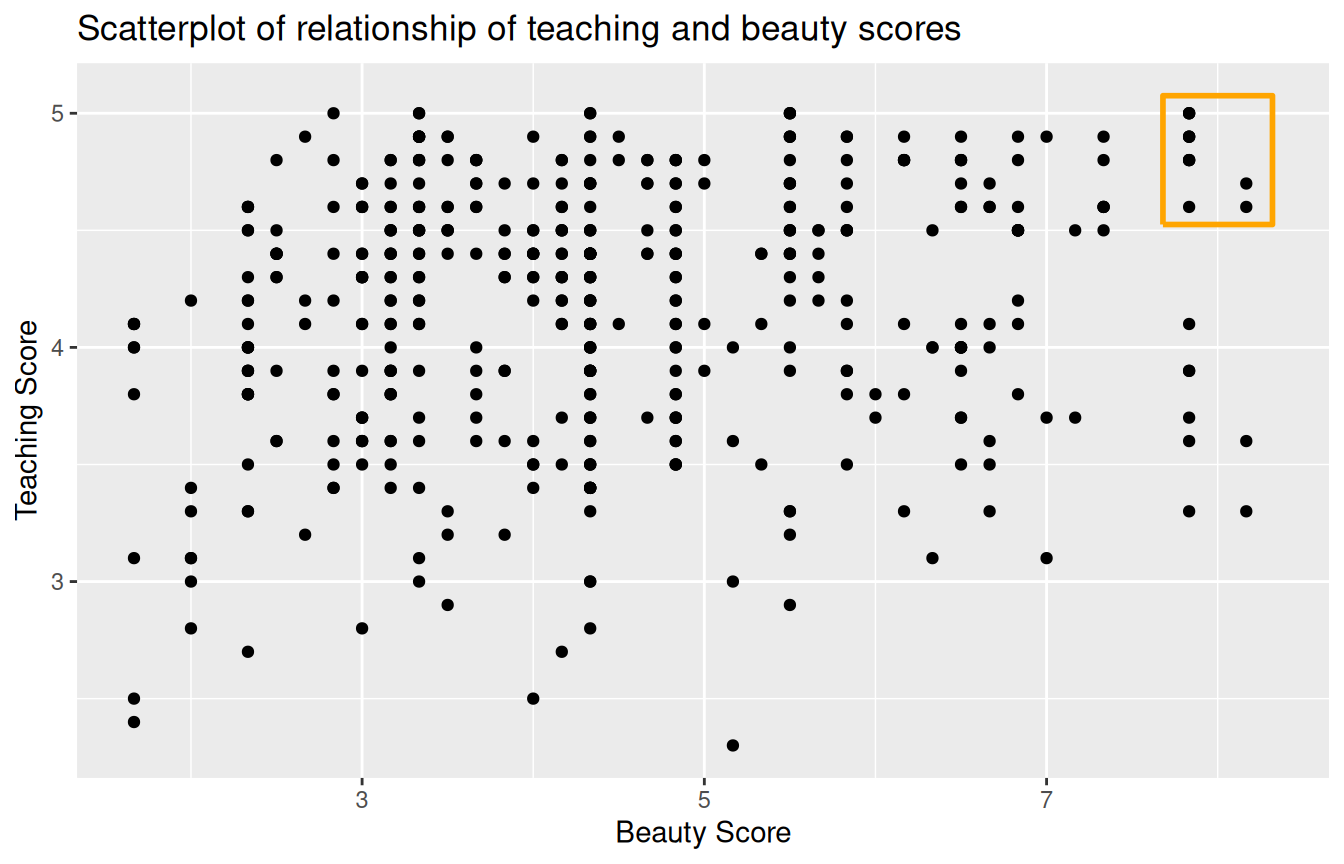FIGURE 5.2: Instructor evaluation scores at UT Austin.

Observe that most “beauty” scores lie between 2 and 8, while most teaching scores lie between 3 and 5. Furthermore, while opinions may vary, it is our opinion that the relationship between teaching score and “beauty” score is “weakly positive.” This is consistent with our earlier computed correlation coefficient of 0.187.

Furthermore, there appear to be six points in the top-right of this plot highlighted in the box. However, this is not actually the case, as this plot suffers from overplotting. Recall from Subsection 2.3.2 that overplotting occurs when several points are stacked directly on top of each other, making it difficult to distinguish them. So while it may appear that there are only six points in the box, there are actually more. This fact is only apparent when using geom_jitter() in place of geom_point(). We display the resulting plot in Figure 5.3 along with the same small box as in Figure 5.2.

ggplot(evals_ch5, aes(x = bty_avg, y = score)) +
geom_jitter() +
labs(x = "Beauty Score", y = "Teaching Score",
title = "Scatterplot of relationship of teaching and beauty scores")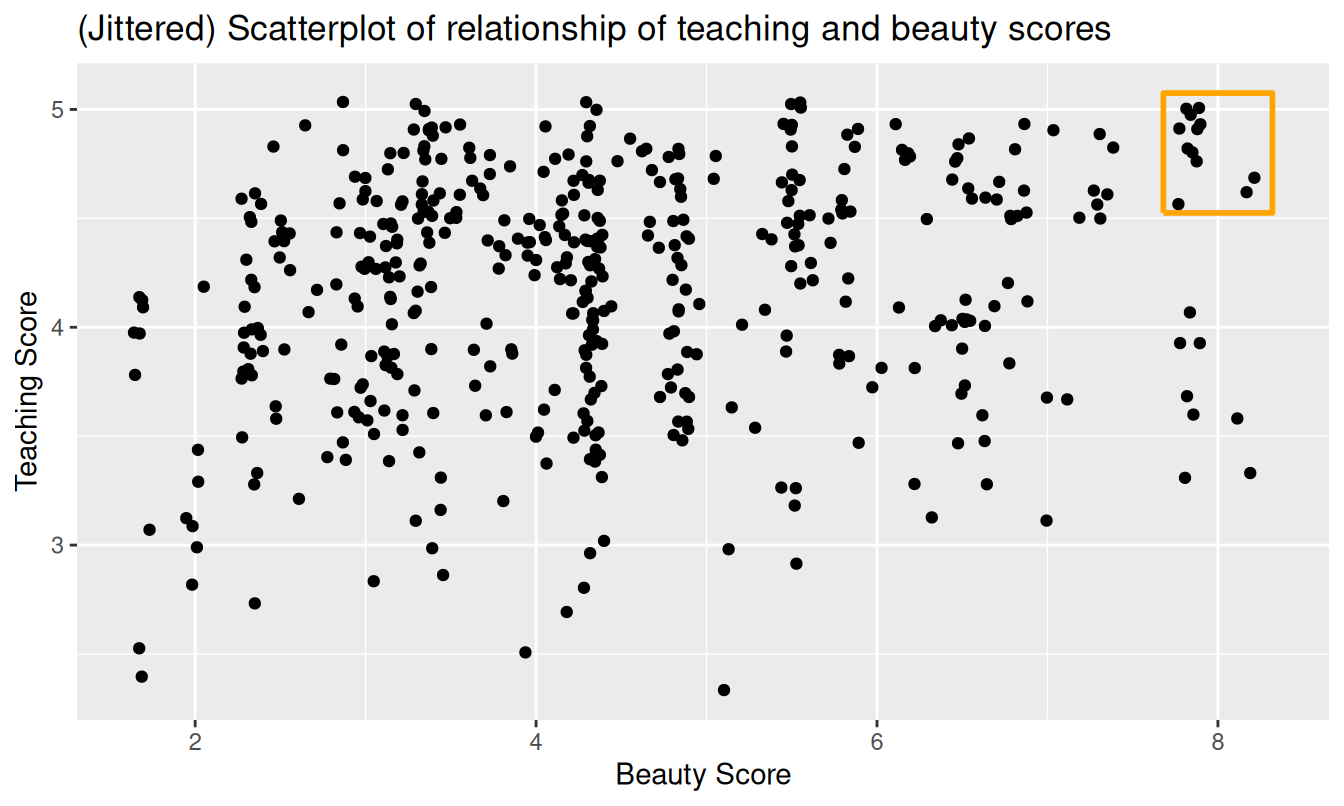FIGURE 5.3: Instructor evaluation scores at UT Austin.

It is now apparent that there are 12 points in the area highlighted in the box and not six as originally suggested in Figure 5.2. Recall from Subsection 2.3.2 on overplotting that jittering adds a little random “nudge” to each of the points to break up these ties. Furthermore, recall that jittering is strictly a visualization tool; it does not alter the original values in the data frame evals_ch5. To keep things simple going forward, however, we’ll only present regular scatterplots rather than their jittered counterparts.

Let’s build on the unjittered scatterplot in Figure 5.2 by adding a “best-fitting” line: of all possible lines we can draw on this scatterplot, it is the line that “best” fits through the cloud of points. We do this by adding a new geom_smooth(method = "lm", se = FALSE) layer to the ggplot() code that created the scatterplot in Figure 5.2. The method = "lm" argument sets the line to be a “linear model.” The se = FALSE argument suppresses standard error uncertainty bars. (We’ll define the concept of standard error later in Subsection 7.3.2.)

ggplot(evals_ch5, aes(x = bty_avg, y = score)) +
geom_point() +
labs(x = "Beauty Score", y = "Teaching Score",
title = "Relationship between teaching and beauty scores") +
geom_smooth(method = "lm", se = FALSE)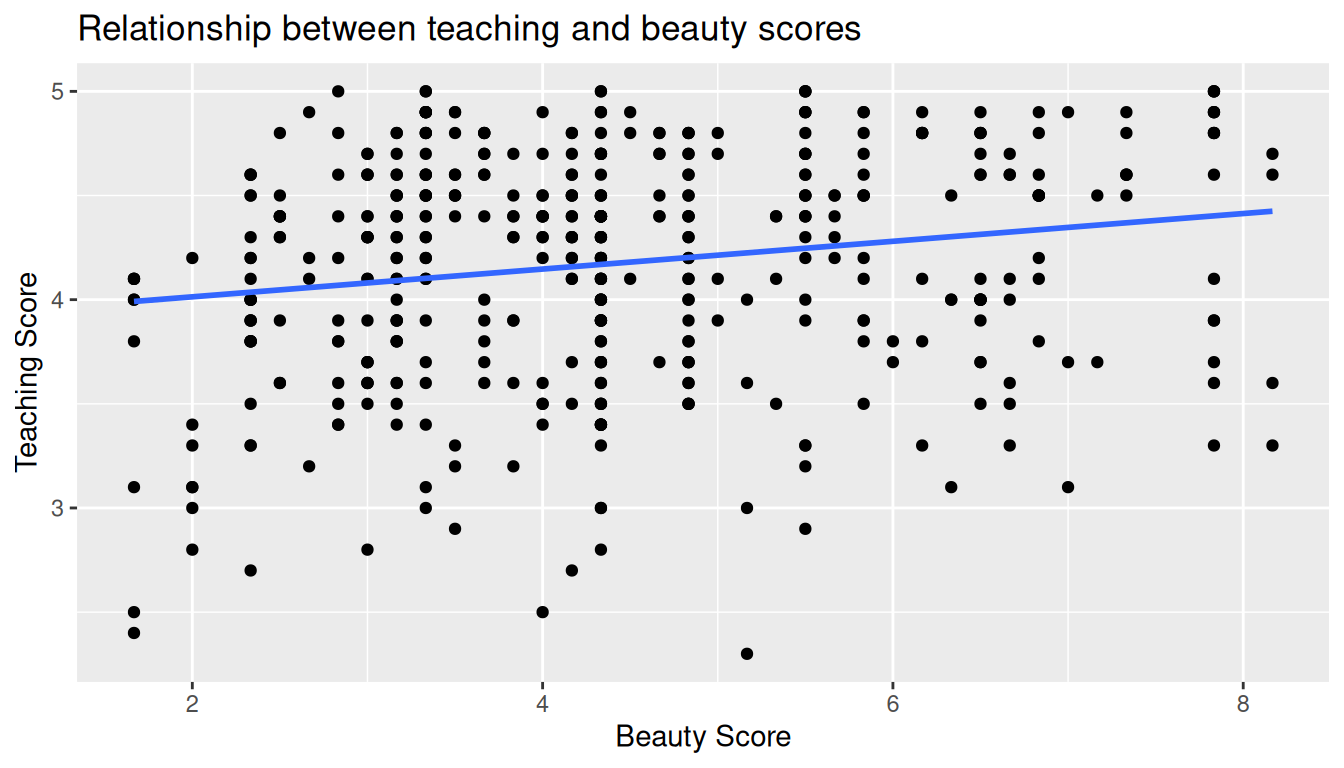FIGURE 5.4: Regression line.

The line in the resulting Figure 5.4 is called a “regression line.” The regression line is a visual summary of the relationship between two numerical variables, in our case the outcome variable score and the explanatory variable bty_avg. The positive slope of the blue line is consistent with our earlier observed correlation coefficient of 0.187 suggesting that there is a positive relationship between these two variables: as instructors have higher “beauty” scores, so also do they receive higher teaching evaluations. We’ll see later, however, that while the correlation coefficient and the slope of a regression line always have the same sign (positive or negative), they typically do not have the same value.

Furthermore, a regression line is “best-fitting” in that it minimizes some mathematical criteria. We present these mathematical criteria in Subsection 5.3.2, but we suggest you read this subsection only after first reading the rest of this section on regression with one numerical explanatory variable.

Learning check

(LC5.1) Conduct a new exploratory data analysis with the same outcome variable $$y$$ being score but with age as the new explanatory variable $$x$$. Remember, this involves three things:

1. Looking at the raw data values.
2. Computing summary statistics.
3. Creating data visualizations.

What can you say about the relationship between age and teaching scores based on this exploration?

### 5.1.2 Simple linear regression

You may recall from secondary/high school algebra that the equation of a line is $$y = a + b\cdot x$$. (Note that the $$\cdot$$ symbol is equivalent to the $$\times$$ “multiply by” mathematical symbol. We’ll use the $$\cdot$$ symbol in the rest of this book as it is more succinct.) It is defined by two coefficients $$a$$ and $$b$$. The intercept coefficient $$a$$ is the value of $$y$$ when $$x = 0$$. The slope coefficient $$b$$ for $$x$$ is the increase in $$y$$ for every increase of one in $$x$$. This is also called the “rise over run.”

However, when defining a regression line like the regression line in Figure 5.4, we use slightly different notation: the equation of the regression line is $$\widehat{y} = b_0 + b_1 \cdot x$$ . The intercept coefficient is $$b_0$$, so $$b_0$$ is the value of $$\widehat{y}$$ when $$x = 0$$. The slope coefficient for $$x$$ is $$b_1$$, i.e., the increase in $$\widehat{y}$$ for every increase of one in $$x$$. Why do we put a “hat” on top of the $$y$$? It’s a form of notation commonly used in regression to indicate that we have a “fitted value,” or the value of $$y$$ on the regression line for a given $$x$$ value. We’ll discuss this more in the upcoming Subsection 5.1.3.

We know that the regression line in Figure 5.4 has a positive slope $$b_1$$ corresponding to our explanatory $$x$$ variable bty_avg. Why? Because as instructors tend to have higher bty_avg scores, so also do they tend to have higher teaching evaluation scores. However, what is the numerical value of the slope $$b_1$$? What about the intercept $$b_0$$? Let’s not compute these two values by hand, but rather let’s use a computer!

We can obtain the values of the intercept $$b_0$$ and the slope for bty_avg $$b_1$$ by outputting a linear regression table. This is done in two steps:

1. We first “fit” the linear regression model using the lm() function and save it in score_model.
2. We get the regression table by applying the get_regression_table() function from the moderndive package to score_model.
# Fit regression model:
score_model <- lm(score ~ bty_avg, data = evals_ch5)
# Get regression table:
get_regression_table(score_model)
TABLE 5.2: Linear regression table
term estimate std_error statistic p_value lower_ci upper_ci
intercept 3.880 0.076 50.96 0 3.731 4.030
bty_avg 0.067 0.016 4.09 0 0.035 0.099

Let’s first focus on interpreting the regression table output in Table 5.2, and then we’ll later revisit the code that produced it. In the estimate column of Table 5.2 are the intercept $$b_0$$ = 3.88 and the slope $$b_1$$ = 0.067 for bty_avg. Thus the equation of the regression line in Figure 5.4 follows:

\begin{aligned} \widehat{y} &= b_0 + b_1 \cdot x\\ \widehat{\text{score}} &= b_0 + b_{\text{bty}\_\text{avg}} \cdot\text{bty}\_\text{avg}\\ &= 3.88 + 0.067\cdot\text{bty}\_\text{avg} \end{aligned}

The intercept $$b_0$$ = 3.88 is the average teaching score $$\widehat{y}$$ = $$\widehat{\text{score}}$$ for those courses where the instructor had a “beauty” score bty_avg of 0. Or in graphical terms, it’s where the line intersects the $$y$$ axis when $$x$$ = 0. Note, however, that while the intercept of the regression line has a mathematical interpretation, it has no practical interpretation here, since observing a bty_avg of 0 is impossible; it is the average of six panelists’ “beauty” scores ranging from 1 to 10. Furthermore, looking at the scatterplot with the regression line in Figure 5.4, no instructors had a “beauty” score anywhere near 0.

Of greater interest is the slope $$b_1$$ = $$b_{\text{bty}\_\text{avg}}$$ for bty_avg of 0.067, as this summarizes the relationship between the teaching and “beauty” score variables. Note that the sign is positive, suggesting a positive relationship between these two variables, meaning teachers with higher “beauty” scores also tend to have higher teaching scores. Recall from earlier that the correlation coefficient is 0.187. They both have the same positive sign, but have a different value. Recall further that the correlation’s interpretation is the “strength of linear association”. The slope’s interpretation is a little different:

For every increase of 1 unit in bty_avg, there is an associated increase of, on average, 0.067 units of score.

We only state that there is an associated increase and not necessarily a causal increase. For example, perhaps it’s not that higher “beauty” scores directly cause higher teaching scores per se. Instead, the following could hold true: individuals from wealthier backgrounds tend to have stronger educational backgrounds and hence have higher teaching scores, while at the same time these wealthy individuals also tend to have higher “beauty” scores. In other words, just because two variables are strongly associated, it doesn’t necessarily mean that one causes the other. This is summed up in the often quoted phrase, “correlation is not necessarily causation.” We discuss this idea further in Subsection 5.3.1.

Furthermore, we say that this associated increase is on average 0.067 units of teaching score, because you might have two instructors whose bty_avg scores differ by 1 unit, but their difference in teaching scores won’t necessarily be exactly 0.067. What the slope of 0.067 is saying is that across all possible courses, the average difference in teaching score between two instructors whose “beauty” scores differ by one is 0.067.

Now that we’ve learned how to compute the equation for the regression line in Figure 5.4 using the values in the estimate column of Table 5.2, and how to interpret the resulting intercept and slope, let’s revisit the code that generated this table:

# Fit regression model:
score_model <- lm(score ~ bty_avg, data = evals_ch5)
# Get regression table:
get_regression_table(score_model)

First, we “fit” the linear regression model to the data using the lm() function and save this as score_model. When we say “fit”, we mean “find the best fitting line to this data.” lm() stands for “linear model” and is used as follows: lm(y ~ x, data = data_frame_name) where:

• y is the outcome variable, followed by a tilde ~. In our case, y is set to score.
• x is the explanatory variable. In our case, x is set to bty_avg.
• The combination of y ~ x is called a model formula. (Note the order of y and x.) In our case, the model formula is score ~ bty_avg. We saw such model formulas earlier when we computed the correlation coefficient using the get_correlation() function in Subsection 5.1.1.
• data_frame_name is the name of the data frame that contains the variables y and x. In our case, data_frame_name is the evals_ch5 data frame.

Second, we take the saved model in score_model and apply the get_regression_table() function from the moderndive package to it to obtain the regression table in Table 5.2. This function is an example of what’s known in computer programming as a wrapper function. They take other pre-existing functions and “wrap” them into a single function that hides its inner workings. This concept is illustrated in Figure 5.5.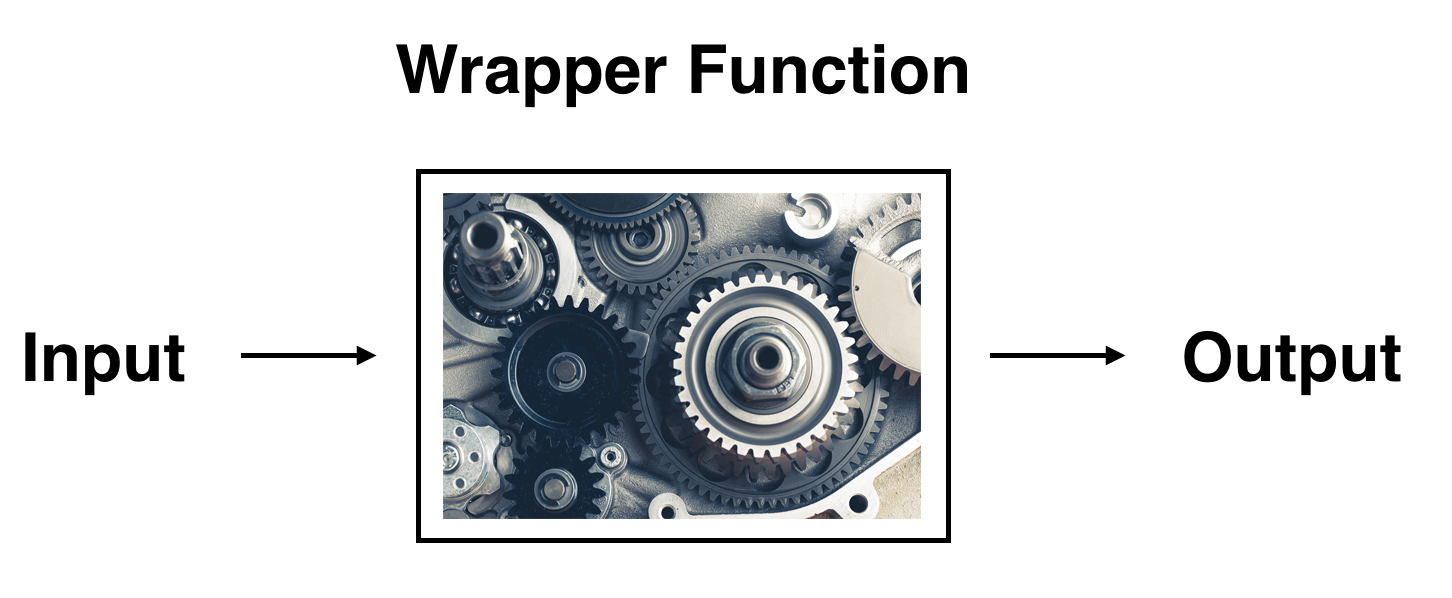FIGURE 5.5: The concept of a wrapper function.

So all you need to worry about is what the inputs look like and what the outputs look like; you leave all the other details “under the hood of the car.” In our regression modeling example, the get_regression_table() function takes a saved lm() linear regression model as input and returns a data frame of the regression table as output. If you’re interested in learning more about the get_regression_table() function’s inner workings, check out Subsection 5.3.3.

Lastly, you might be wondering what the remaining five columns in Table 5.2 are: std_error, statistic, p_value, lower_ci and upper_ci. They are the standard error, test statistic, p-value, lower 95% confidence interval bound, and upper 95% confidence interval bound. They tell us about both the statistical significance and practical significance of our results. This is loosely the “meaningfulness” of our results from a statistical perspective. Let’s put aside these ideas for now and revisit them in Chapter 10 on (statistical) inference for regression. We’ll do this after we’ve had a chance to cover standard errors in Chapter 7, confidence intervals in Chapter 8, and hypothesis testing and $$p$$-values in Chapter 9.

Learning check

(LC5.2) Fit a new simple linear regression using lm(score ~ age, data = evals_ch5) where age is the new explanatory variable $$x$$. Get information about the “best-fitting” line from the regression table by applying the get_regression_table() function. How do the regression results match up with the results from your earlier exploratory data analysis?

### 5.1.3 Observed/fitted values and residuals

We just saw how to get the value of the intercept and the slope of a regression line from the estimate column of a regression table generated by the get_regression_table() function. Now instead say we want information on individual observations. For example, let’s focus on the 21st of the 463 courses in the evals_ch5 data frame in Table 5.3:

TABLE 5.3: Data for the 21st course out of 463
ID score bty_avg age
21 4.9 7.33 31

What is the value $$\widehat{y}$$ on the regression line corresponding to this instructor’s bty_avg “beauty” score of 7.333? In Figure 5.6 we mark three values corresponding to the instructor for this 21st course and give their statistical names:

• Circle: The observed value $$y$$ = 4.9 is this course’s instructor’s actual teaching score.
• Square: The fitted value $$\widehat{y}$$ is the value on the regression line for $$x$$ = bty_avg = 7.333. This value is computed using the intercept and slope in the previous regression table:

$\widehat{y} = b_0 + b_1 \cdot x = 3.88 + 0.067 \cdot 7.333 = 4.369$

• Arrow: The length of this arrow is the residual and is computed by subtracting the fitted value $$\widehat{y}$$ from the observed value $$y$$. The residual can be thought of as a model’s error or “lack of fit” for a particular observation. In the case of this course’s instructor, it is $$y - \widehat{y}$$ = 4.9 - 4.369 = 0.531.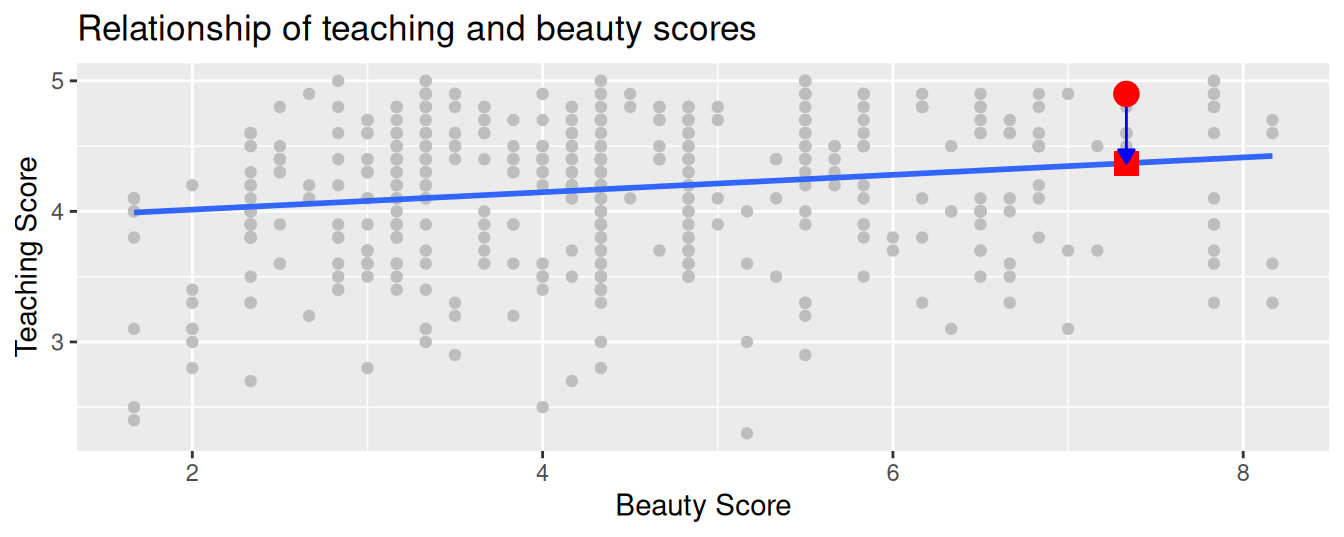FIGURE 5.6: Example of observed value, fitted value, and residual.

Now say we want to compute both the fitted value $$\widehat{y} = b_0 + b_1 \cdot x$$ and the residual $$y - \widehat{y}$$ for all 463 courses in the study. Recall that each course corresponds to one of the 463 rows in the evals_ch5 data frame and also one of the 463 points in the regression plot in Figure 5.6.

We could repeat the previous calculations we performed by hand 463 times, but that would be tedious and time consuming. Instead, let’s do this using a computer with the get_regression_points() function. Just like the get_regression_table() function, the get_regression_points() function is a “wrapper” function. However, this function returns a different output. Let’s apply the get_regression_points() function to score_model, which is where we saved our lm() model in the previous section. In Table 5.4 we present the results of only the 21st through 24th courses for brevity’s sake.

regression_points <- get_regression_points(score_model)
regression_points
TABLE 5.4: Regression points (for only the 21st through 24th courses)
ID score bty_avg score_hat residual
21 4.9 7.33 4.37 0.531
22 4.6 7.33 4.37 0.231
23 4.5 7.33 4.37 0.131
24 4.4 5.50 4.25 0.153

Let’s inspect the individual columns and match them with the elements of Figure 5.6:

• The score column represents the observed outcome variable $$y$$. This is the y-position of the 463 black points.
• The bty_avg column represents the values of the explanatory variable $$x$$. This is the x-position of the 463 black points.
• The score_hat column represents the fitted values $$\widehat{y}$$. This is the corresponding value on the regression line for the 463 $$x$$ values.
• The residual column represents the residuals $$y - \widehat{y}$$. This is the 463 vertical distances between the 463 black points and the regression line.

Just as we did for the instructor of the 21st course in the evals_ch5 dataset (in the first row of the table), let’s repeat the calculations for the instructor of the 24th course (in the fourth row of Table 5.4):

• score = 4.4 is the observed teaching score $$y$$ for this course’s instructor.
• bty_avg = 5.50 is the value of the explanatory variable bty_avg $$x$$ for this course’s instructor.
• score_hat = 4.25 = 3.88 + 0.067 $$\cdot$$ 5.50 is the fitted value $$\widehat{y}$$ on the regression line for this course’s instructor.
• residual = 0.153 = 4.4 - 4.25 is the value of the residual for this instructor. In other words, the model’s fitted value was off by 0.153 teaching score units for this course’s instructor.

At this point, you can skip ahead if you like to Subsection 5.3.2 to learn about the processes behind what makes “best-fitting” regression lines. As a primer, a “best-fitting” line refers to the line that minimizes the sum of squared residuals out of all possible lines we can draw through the points. In Section 5.2, we’ll discuss another common scenario of having a categorical explanatory variable and a numerical outcome variable.

Learning check

(LC5.3) Generate a data frame of the residuals of the model where you used age as the explanatory $$x$$ variable.

## 5.2 One categorical explanatory variable

It’s an unfortunate truth that life expectancy is not the same across all countries in the world. International development agencies are interested in studying these differences in life expectancy in the hopes of identifying where governments should allocate resources to address this problem. In this section, we’ll explore differences in life expectancy in two ways:

1. Differences between continents: Are there significant differences in average life expectancy between the five populated continents of the world: Africa, the Americas, Asia, Europe, and Oceania?
2. Differences within continents: How does life expectancy vary within the world’s five continents? For example, is the spread of life expectancy among the countries of Africa larger than the spread of life expectancy among the countries of Asia?

To answer such questions, we’ll use the gapminder data frame included in the gapminder package. This dataset has international development statistics such as life expectancy, GDP per capita, and population for 142 countries for 5-year intervals between 1952 and 2007. Recall we visualized some of this data in Figure 2.1 in Subsection 2.1.2 on the grammar of graphics.

We’ll use this data for basic regression again, but now using an explanatory variable $$x$$ that is categorical, as opposed to the numerical explanatory variable model we used in the previous Section 5.1.

1. A numerical outcome variable $$y$$ (a country’s life expectancy) and
2. A single categorical explanatory variable $$x$$ (the continent that the country is a part of).

When the explanatory variable $$x$$ is categorical, the concept of a “best-fitting” regression line is a little different than the one we saw previously in Section 5.1 where the explanatory variable $$x$$ was numerical. We’ll study these differences shortly in Subsection 5.2.2, but first we conduct an exploratory data analysis.

### 5.2.1 Exploratory data analysis

The data on the 142 countries can be found in the gapminder data frame included in the gapminder package. However, to keep things simple, let’s filter() for only those observations/rows corresponding to the year 2007. Additionally, let’s select() only the subset of the variables we’ll consider in this chapter. We’ll save this data in a new data frame called gapminder2007:

library(gapminder)
gapminder2007 <- gapminder %>%
filter(year == 2007) %>%
select(country, lifeExp, continent, gdpPercap)

Let’s perform the first common step in an exploratory data analysis: looking at the raw data values. You can do this by using RStudio’s spreadsheet viewer or by using the glimpse() command as introduced in Subsection 1.4.3 on exploring data frames:

glimpse(gapminder2007)
Rows: 142
Columns: 4
$country <fct> "Afghanistan", "Albania", "Algeria", "Angola", "Argentina", …$ lifeExp   <dbl> 43.8, 76.4, 72.3, 42.7, 75.3, 81.2, 79.8, 75.6, 64.1, 79.4, …
$continent <fct> Asia, Europe, Africa, Africa, Americas, Oceania, Europe, Asi…$ gdpPercap <dbl> 975, 5937, 6223, 4797, 12779, 34435, 36126, 29796, 1391, 336…

Observe that Rows: 142 indicates that there are 142 rows/observations in gapminder2007, where each row corresponds to one country. In other words, the observational unit is an individual country. Furthermore, observe that the variable continent is of type <fct>, which stands for factor, which is R’s way of encoding categorical variables.

A full description of all the variables included in gapminder can be found by reading the associated help file (run ?gapminder in the console). However, let’s fully describe only the 4 variables we selected in gapminder2007:

1. country: An identification variable of type character/text used to distinguish the 142 countries in the dataset.
2. lifeExp: A numerical variable of that country’s life expectancy at birth. This is the outcome variable $$y$$ of interest.
3. continent: A categorical variable with five levels. Here “levels” correspond to the possible categories: Africa, Asia, Americas, Europe, and Oceania. This is the explanatory variable $$x$$ of interest.
4. gdpPercap: A numerical variable of that country’s GDP per capita in US inflation-adjusted dollars that we’ll use as another outcome variable $$y$$ in the Learning check at the end of this subsection.

Let’s look at a random sample of five out of the 142 countries in Table 5.5.

gapminder2007 %>% sample_n(size = 5)
TABLE 5.5: Random sample of 5 out of 142 countries
country lifeExp continent gdpPercap
Togo 58.4 Africa 883
Sao Tome and Principe 65.5 Africa 1598
Congo, Dem. Rep.  46.5 Africa 278
Lesotho 42.6 Africa 1569
Bulgaria 73.0 Europe 10681

Note that random sampling will likely produce a different subset of 5 rows for you than what’s shown. Now that we’ve looked at the raw values in our gapminder2007 data frame and got a sense of the data, let’s move on to computing summary statistics. Let’s once again apply the skim() function from the skimr package. Recall from our previous EDA that this function takes in a data frame, “skims” it, and returns commonly used summary statistics. Let’s take our gapminder2007 data frame, select() only the outcome and explanatory variables lifeExp and continent, and pipe them into the skim() function:

gapminder2007 %>%
select(lifeExp, continent) %>%
skim()
Skim summary statistics
n obs: 142
n variables: 2

── Variable type:factor
variable missing complete   n n_unique                         top_counts ordered
continent       0      142 142        5 Afr: 52, Asi: 33, Eur: 30, Ame: 25   FALSE

── Variable type:numeric
variable missing complete   n  mean    sd    p0   p25   p50   p75 p100
lifeExp       0      142 142 67.01 12.07 39.61 57.16 71.94 76.41 82.6

The skim() output now reports summaries for categorical variables (Variable type:factor) separately from the numerical variables (Variable type:numeric). For the categorical variable continent, it reports:

• missing, complete, and n, which are the number of missing, complete, and total number of values as before, respectively.
• n_unique: The number of unique levels to this variable, corresponding to Africa, Asia, Americas, Europe, and Oceania. This refers to how many countries are in the data for each continent.
• top_counts: In this case, the top four counts: Africa has 52 countries, Asia has 33, Europe has 30, and Americas has 25. Not displayed is Oceania with 2 countries.
• ordered: This tells us whether the categorical variable is “ordinal”: whether there is an encoded hierarchy (like low, medium, high). In this case, continent is not ordered.

Turning our attention to the summary statistics of the numerical variable lifeExp, we observe that the global median life expectancy in 2007 was 71.94. Thus, half of the world’s countries (71 countries) had a life expectancy less than 71.94. The mean life expectancy of 67.01 is lower, however. Why is the mean life expectancy lower than the median?

We can answer this question by performing the last of the three common steps in an exploratory data analysis: creating data visualizations. Let’s visualize the distribution of our outcome variable $$y$$ = lifeExp in Figure 5.7.

ggplot(gapminder2007, aes(x = lifeExp)) +
geom_histogram(binwidth = 5, color = "white") +
labs(x = "Life expectancy", y = "Number of countries",
title = "Histogram of distribution of worldwide life expectancies")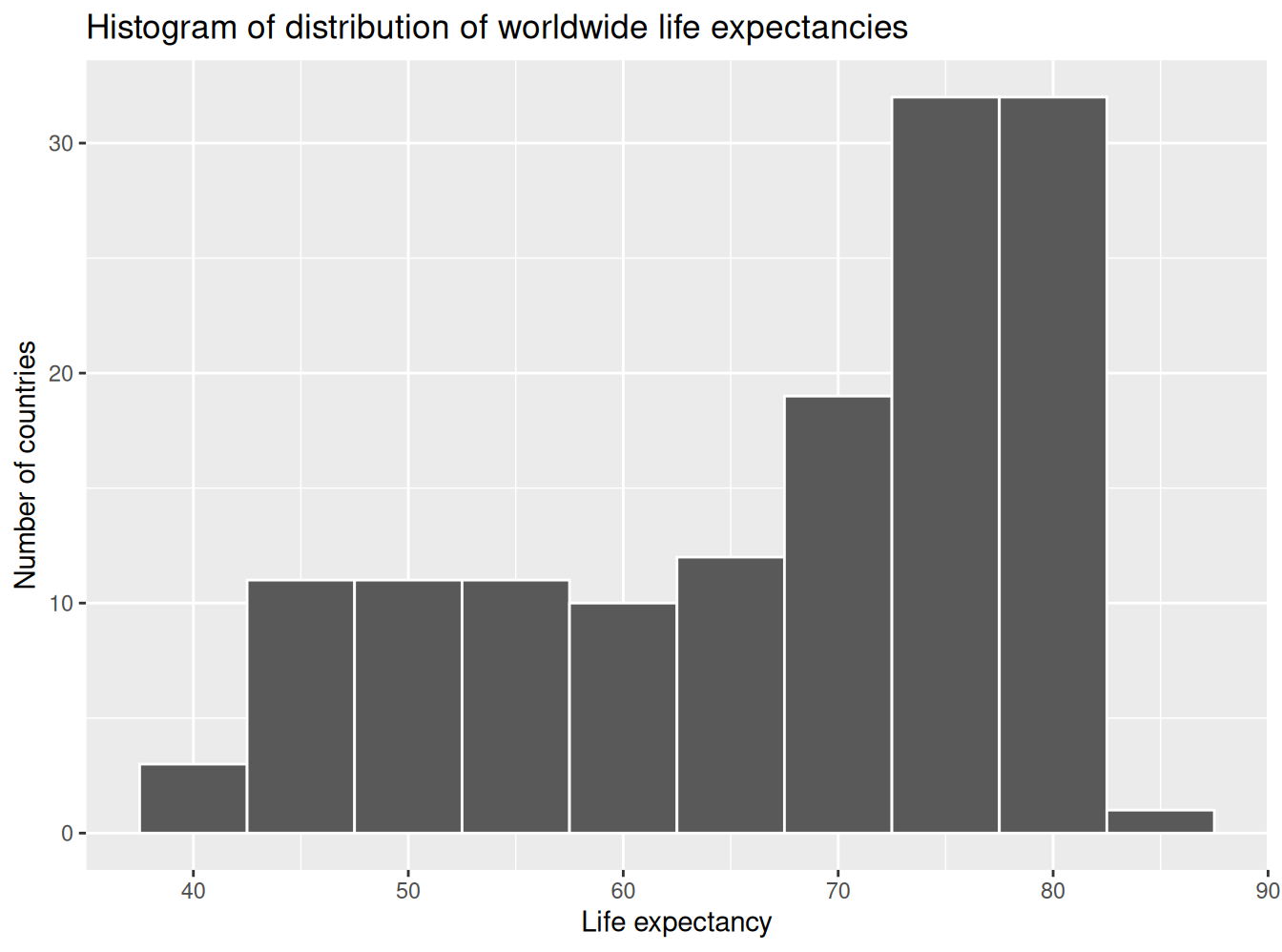FIGURE 5.7: Histogram of life expectancy in 2007.

We see that this data is left-skewed, also known as negatively skewed: there are a few countries with low life expectancy that are bringing down the mean life expectancy. However, the median is less sensitive to the effects of such outliers; hence, the median is greater than the mean in this case.

Remember, however, that we want to compare life expectancies both between continents and within continents. In other words, our visualizations need to incorporate some notion of the variable continent. We can do this easily with a faceted histogram. Recall from Section 2.6 that facets allow us to split a visualization by the different values of another variable. We display the resulting visualization in Figure 5.8 by adding a facet_wrap(~ continent, nrow = 2) layer.

ggplot(gapminder2007, aes(x = lifeExp)) +
geom_histogram(binwidth = 5, color = "white") +
labs(x = "Life expectancy",
y = "Number of countries",
title = "Histogram of distribution of worldwide life expectancies") +
facet_wrap(~ continent, nrow = 2)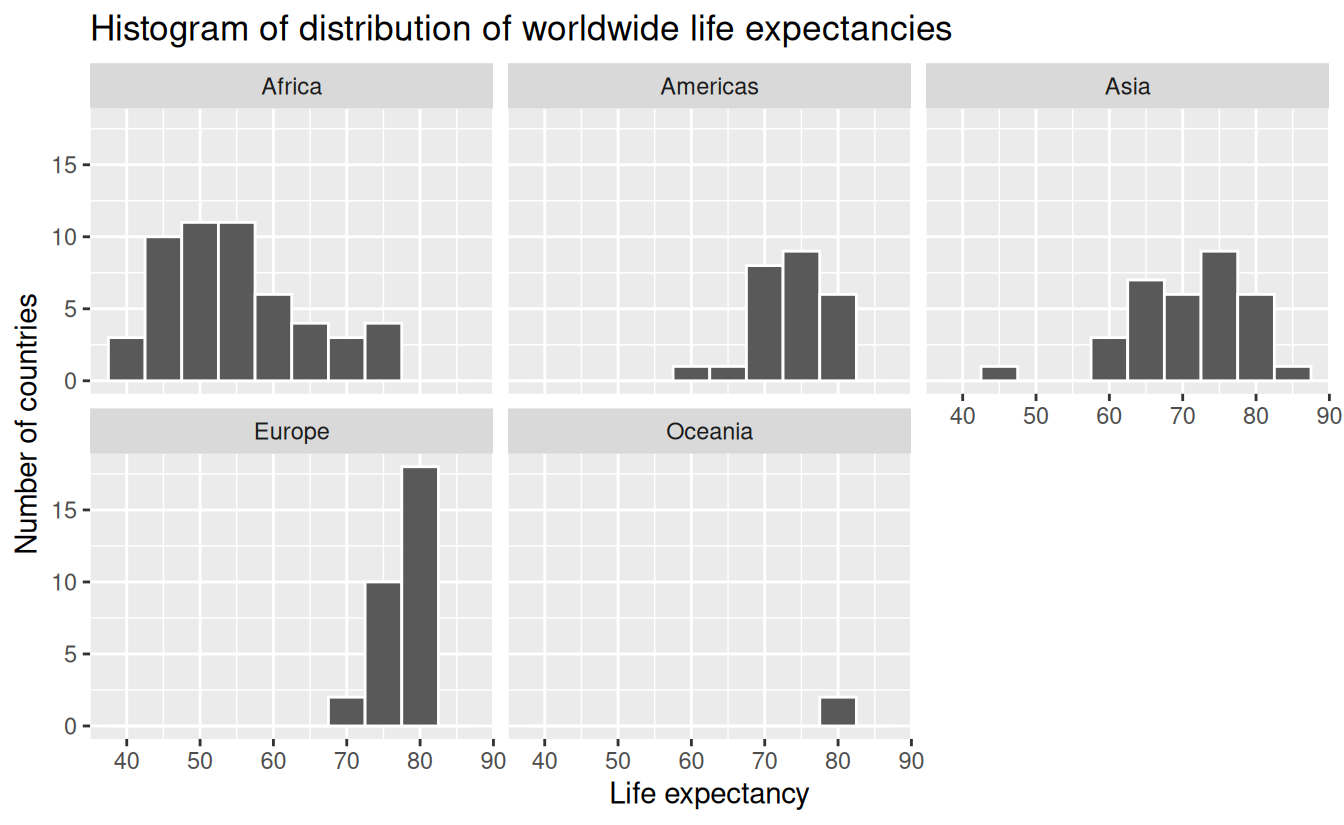FIGURE 5.8: Life expectancy in 2007.

Observe that unfortunately the distribution of African life expectancies is much lower than the other continents, while in Europe life expectancies tend to be higher and furthermore do not vary as much. On the other hand, both Asia and Africa have the most variation in life expectancies. There is the least variation in Oceania, but keep in mind that there are only two countries in Oceania: Australia and New Zealand.

Recall that an alternative method to visualize the distribution of a numerical variable split by a categorical variable is by using a side-by-side boxplot. We map the categorical variable continent to the $$x$$-axis and the different life expectancies within each continent on the $$y$$-axis in Figure 5.9.

ggplot(gapminder2007, aes(x = continent, y = lifeExp)) +
geom_boxplot() +
labs(x = "Continent", y = "Life expectancy",
title = "Life expectancy by continent")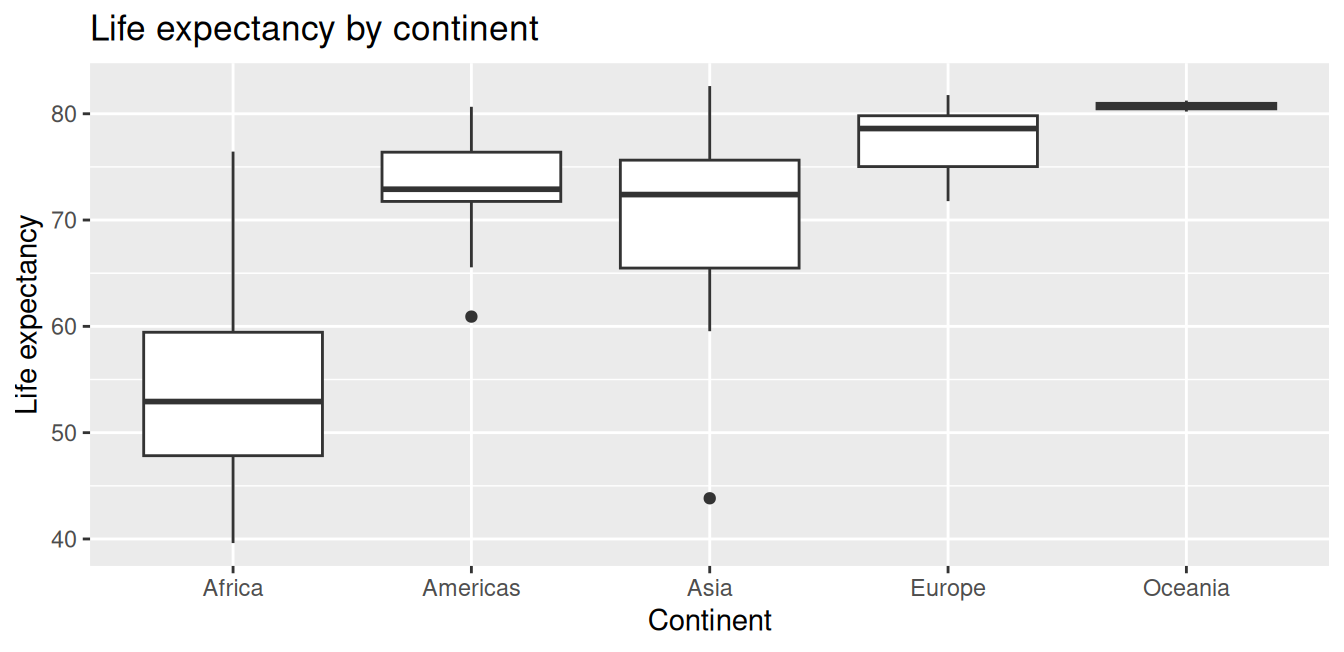FIGURE 5.9: Life expectancy in 2007.

Some people prefer comparing the distributions of a numerical variable between different levels of a categorical variable using a boxplot instead of a faceted histogram. This is because we can make quick comparisons between the categorical variable’s levels with imaginary horizontal lines. For example, observe in Figure 5.9 that we can quickly convince ourselves that Oceania has the highest median life expectancies by drawing an imaginary horizontal line at $$y$$ = 80. Furthermore, as we observed in the faceted histogram in Figure 5.8, Africa and Asia have the largest variation in life expectancy as evidenced by their large interquartile ranges (the heights of the boxes).

It’s important to remember, however, that the solid lines in the middle of the boxes correspond to the medians (the middle value) rather than the mean (the average). So, for example, if you look at Asia, the solid line denotes the median life expectancy of around 72 years. This tells us that half of all countries in Asia have a life expectancy below 72 years, whereas half have a life expectancy above 72 years.

Let’s compute the median and mean life expectancy for each continent with a little more data wrangling and display the results in Table 5.6.

lifeExp_by_continent <- gapminder2007 %>%
group_by(continent) %>%
summarize(median = median(lifeExp),
mean = mean(lifeExp))
TABLE 5.6: Life expectancy by continent
continent median mean
Africa 52.9 54.8
Americas 72.9 73.6
Asia 72.4 70.7
Europe 78.6 77.6
Oceania 80.7 80.7

Observe the order of the second column median life expectancy: Africa is lowest, the Americas and Asia are next with similar medians, then Europe, then Oceania. This ordering corresponds to the ordering of the solid black lines inside the boxes in our side-by-side boxplot in Figure 5.9.

Let’s now turn our attention to the values in the third column mean. Using Africa’s mean life expectancy of 54.8 as a baseline for comparison, let’s start making comparisons to the mean life expectancies of the other four continents and put these values in Table 5.7, which we’ll revisit later on in this section.

1. For the Americas, it is 73.6 - 54.8 = 18.8 years higher.
2. For Asia, it is 70.7 - 54.8 = 15.9 years higher.
3. For Europe, it is 77.6 - 54.8 = 22.8 years higher.
4. For Oceania, it is 80.7 - 54.8 = 25.9 years higher.
TABLE 5.7: Mean life expectancy by continent and relative differences from mean for Africa
continent mean Difference versus Africa
Africa 54.8 0.0
Americas 73.6 18.8
Asia 70.7 15.9
Europe 77.6 22.8
Oceania 80.7 25.9

Learning check

(LC5.4) Conduct a new exploratory data analysis with the same explanatory variable $$x$$ being continent but with gdpPercap as the new outcome variable $$y$$. What can you say about the differences in GDP per capita between continents based on this exploration?

### 5.2.2 Linear regression

In Subsection 5.1.2 we introduced simple linear regression, which involves modeling the relationship between a numerical outcome variable $$y$$ and a numerical explanatory variable $$x$$. In our life expectancy example, we now instead have a categorical explanatory variable continent. Our model will not yield a “best-fitting” regression line like in Figure 5.4, but rather offsets relative to a baseline for comparison.

As we did in Subsection 5.1.2 when studying the relationship between teaching scores and “beauty” scores, let’s output the regression table for this model. Recall that this is done in two steps:

1. We first “fit” the linear regression model using the lm(y ~ x, data) function and save it in lifeExp_model.
2. We get the regression table by applying the get_regression_table() function from the moderndive package to lifeExp_model.
lifeExp_model <- lm(lifeExp ~ continent, data = gapminder2007)
get_regression_table(lifeExp_model)
TABLE 5.8: Linear regression table
term estimate std_error statistic p_value lower_ci upper_ci
intercept 54.8 1.02 53.45 0 52.8 56.8
continent: Americas 18.8 1.80 10.45 0 15.2 22.4
continent: Asia 15.9 1.65 9.68 0 12.7 19.2
continent: Europe 22.8 1.70 13.47 0 19.5 26.2
continent: Oceania 25.9 5.33 4.86 0 15.4 36.5

Let’s once again focus on the values in the term and estimate columns of Table 5.8. Why are there now 5 rows? Let’s break them down one-by-one:

1. intercept corresponds to the mean life expectancy of countries in Africa of 54.8 years.
2. continent: Americas corresponds to countries in the Americas and the value +18.8 is the same difference in mean life expectancy relative to Africa we displayed in Table 5.7. In other words, the mean life expectancy of countries in the Americas is $$54.8 + 18.8 = 73.6$$.
3. continent: Asia corresponds to countries in Asia and the value +15.9 is the same difference in mean life expectancy relative to Africa we displayed in Table 5.7. In other words, the mean life expectancy of countries in Asia is $$54.8 + 15.9 = 70.7$$.
4. continent: Europe corresponds to countries in Europe and the value +22.8 is the same difference in mean life expectancy relative to Africa we displayed in Table 5.7. In other words, the mean life expectancy of countries in Europe is $$54.8 + 22.8 = 77.6$$.
5. continent: Oceania corresponds to countries in Oceania and the value +25.9 is the same difference in mean life expectancy relative to Africa we displayed in Table 5.7. In other words, the mean life expectancy of countries in Oceania is $$54.8 + 25.9 = 80.7$$.

To summarize, the 5 values in the estimate column in Table 5.8 correspond to the “baseline for comparison” continent Africa (the intercept) as well as four “offsets” from this baseline for the remaining 4 continents: the Americas, Asia, Europe, and Oceania.

You might be asking at this point why was Africa chosen as the “baseline for comparison” group. This is the case for no other reason than it comes first alphabetically of the five continents; by default R arranges factors/categorical variables in alphanumeric order. You can change this baseline group to be another continent if you manipulate the variable continent’s factor “levels” using the forcats package. See Chapter 15 of R for Data Science for examples.

Let’s now write the equation for our fitted values $$\widehat{y} = \widehat{\text{life exp}}$$.

\begin{aligned} \widehat{y} = \widehat{\text{life exp}} &= b_0 + b_{\text{Amer}}\cdot\mathbb{1}_{\text{Amer}}(x) + b_{\text{Asia}}\cdot\mathbb{1}_{\text{Asia}}(x) + \\ & \qquad b_{\text{Euro}}\cdot\mathbb{1}_{\text{Euro}}(x) + b_{\text{Ocean}}\cdot\mathbb{1}_{\text{Ocean}}(x)\\ &= 54.8 + 18.8\cdot\mathbb{1}_{\text{Amer}}(x) + 15.9\cdot\mathbb{1}_{\text{Asia}}(x) + \\ & \qquad 22.8\cdot\mathbb{1}_{\text{Euro}}(x) + 25.9\cdot\mathbb{1}_{\text{Ocean}}(x) \end{aligned}

Whoa! That looks daunting! Don’t fret, however, as once you understand what all the elements mean, things simplify greatly. First, $$\mathbb{1}_{A}(x)$$ is what’s known in mathematics as an “indicator function.” It returns only one of two possible values, 0 and 1, where

$\mathbb{1}_{A}(x) = \left\{ \begin{array}{ll} 1 & \text{if } x \text{ is in } A \\ 0 & \text{if } \text{otherwise} \end{array} \right.$

In a statistical modeling context, this is also known as a dummy variable. In our case, let’s consider the first such indicator variable $$\mathbb{1}_{\text{Amer}}(x)$$. This indicator function returns 1 if a country is in the Americas, 0 otherwise:

$\mathbb{1}_{\text{Amer}}(x) = \left\{ \begin{array}{ll} 1 & \text{if } \text{country } x \text{ is in the Americas} \\ 0 & \text{otherwise}\end{array} \right.$

Second, $$b_0$$ corresponds to the intercept as before; in this case, it’s the mean life expectancy of all countries in Africa. Third, the $$b_{\text{Amer}}$$, $$b_{\text{Asia}}$$, $$b_{\text{Euro}}$$, and $$b_{\text{Ocean}}$$ represent the 4 “offsets relative to the baseline for comparison” in the regression table output in Table 5.8: continent: Americas, continent: Asia, continent: Europe, and continent: Oceania.

Let’s put this all together and compute the fitted value $$\widehat{y} = \widehat{\text{life exp}}$$ for a country in Africa. Since the country is in Africa, all four indicator functions $$\mathbb{1}_{\text{Amer}}(x)$$, $$\mathbb{1}_{\text{Asia}}(x)$$, $$\mathbb{1}_{\text{Euro}}(x)$$, and $$\mathbb{1}_{\text{Ocean}}(x)$$ will equal 0, and thus:

\begin{aligned} \widehat{\text{life exp}} &= b_0 + b_{\text{Amer}}\cdot\mathbb{1}_{\text{Amer}}(x) + b_{\text{Asia}}\cdot\mathbb{1}_{\text{Asia}}(x) + \\ & \qquad b_{\text{Euro}}\cdot\mathbb{1}_{\text{Euro}}(x) + b_{\text{Ocean}}\cdot\mathbb{1}_{\text{Ocean}}(x)\\ &= 54.8 + 18.8\cdot\mathbb{1}_{\text{Amer}}(x) + 15.9\cdot\mathbb{1}_{\text{Asia}}(x) + \\ & \qquad 22.8\cdot\mathbb{1}_{\text{Euro}}(x) + 25.9\cdot\mathbb{1}_{\text{Ocean}}(x)\\ &= 54.8 + 18.8\cdot 0 + 15.9\cdot 0 + 22.8\cdot 0 + 25.9\cdot 0\\ &= 54.8 \end{aligned}

In other words, all that’s left is the intercept $$b_0$$, corresponding to the average life expectancy of African countries of 54.8 years. Next, say we are considering a country in the Americas. In this case, only the indicator function $$\mathbb{1}_{\text{Amer}}(x)$$ for the Americas will equal 1, while all the others will equal 0, and thus:

\begin{aligned} \widehat{\text{life exp}} &= 54.8 + 18.8\cdot\mathbb{1}_{\text{Amer}}(x) + 15.9\cdot\mathbb{1}_{\text{Asia}}(x) + 22.8\cdot\mathbb{1}_{\text{Euro}}(x) + \\ & \qquad 25.9\cdot\mathbb{1}_{\text{Ocean}}(x)\\ &= 54.8 + 18.8\cdot 1 + 15.9\cdot 0 + 22.8\cdot 0 + 25.9\cdot 0\\ &= 54.8 + 18.8 \\ & = 73.6 \end{aligned}

which is the mean life expectancy for countries in the Americas of 73.6 years in Table 5.7. Note the “offset from the baseline for comparison” is +18.8 years.

Let’s do one more. Say we are considering a country in Asia. In this case, only the indicator function $$\mathbb{1}_{\text{Asia}}(x)$$ for Asia will equal 1, while all the others will equal 0, and thus:

\begin{aligned} \widehat{\text{life exp}} &= 54.8 + 18.8\cdot\mathbb{1}_{\text{Amer}}(x) + 15.9\cdot\mathbb{1}_{\text{Asia}}(x) + 22.8\cdot\mathbb{1}_{\text{Euro}}(x) + \\ & \qquad 25.9\cdot\mathbb{1}_{\text{Ocean}}(x)\\ &= 54.8 + 18.8\cdot 0 + 15.9\cdot 1 + 22.8\cdot 0 + 25.9\cdot 0\\ &= 54.8 + 15.9 \\ & = 70.7 \end{aligned}

which is the mean life expectancy for Asian countries of 70.7 years in Table 5.7. The “offset from the baseline for comparison” here is +15.9 years.

Let’s generalize this idea a bit. If we fit a linear regression model using a categorical explanatory variable $$x$$ that has $$k$$ possible categories, the regression table will return an intercept and $$k - 1$$ “offsets.” In our case, since there are $$k = 5$$ continents, the regression model returns an intercept corresponding to the baseline for comparison group of Africa and $$k - 1 = 4$$ offsets corresponding to the Americas, Asia, Europe, and Oceania.

Understanding a regression table output when you’re using a categorical explanatory variable is a topic those new to regression often struggle with. The only real remedy for these struggles is practice, practice, practice. However, once you equip yourselves with an understanding of how to create regression models using categorical explanatory variables, you’ll be able to incorporate many new variables into your models, given the large amount of the world’s data that is categorical. If you feel like you’re still struggling at this point, however, we suggest you closely compare Tables 5.7 and 5.8 and note how you can compute all the values from one table using the values in the other.

Learning check

(LC5.5) Fit a new linear regression using lm(gdpPercap ~ continent, data = gapminder2007) where gdpPercap is the new outcome variable $$y$$. Get information about the “best-fitting” line from the regression table by applying the get_regression_table() function. How do the regression results match up with the results from your previous exploratory data analysis?

### 5.2.3 Observed/fitted values and residuals

Recall in Subsection 5.1.3, we defined the following three concepts:

1. Observed values $$y$$, or the observed value of the outcome variable
2. Fitted values $$\widehat{y}$$, or the value on the regression line for a given $$x$$ value
3. Residuals $$y - \widehat{y}$$, or the error between the observed value and the fitted value

We obtained these values and other values using the get_regression_points() function from the moderndive package. This time, however, let’s add an argument setting ID = "country": this is telling the function to use the variable country in gapminder2007 as an identification variable in the output. This will help contextualize our analysis by matching values to countries.

regression_points <- get_regression_points(lifeExp_model, ID = "country")
regression_points
TABLE 5.9: Regression points (First 10 out of 142 countries)
country lifeExp continent lifeExp_hat residual
Afghanistan 43.8 Asia 70.7 -26.900
Albania 76.4 Europe 77.6 -1.226
Algeria 72.3 Africa 54.8 17.495
Angola 42.7 Africa 54.8 -12.075
Argentina 75.3 Americas 73.6 1.712
Australia 81.2 Oceania 80.7 0.516
Austria 79.8 Europe 77.6 2.180
Bahrain 75.6 Asia 70.7 4.907
Belgium 79.4 Europe 77.6 1.792

Observe in Table 5.9 that lifeExp_hat contains the fitted values $$\widehat{y}$$ = $$\widehat{\text{lifeExp}}$$. If you look closely, there are only 5 possible values for lifeExp_hat. These correspond to the five mean life expectancies for the 5 continents that we displayed in Table 5.7 and computed using the values in the estimate column of the regression table in Table 5.8.

The residual column is simply $$y - \widehat{y}$$ = lifeExp - lifeExp_hat. These values can be interpreted as the deviation of a country’s life expectancy from its continent’s average life expectancy. For example, look at the first row of Table 5.9 corresponding to Afghanistan. The residual of $$y - \widehat{y} = 43.8 - 70.7 = -26.9$$ is telling us that Afghanistan’s life expectancy is a whopping 26.9 years lower than the mean life expectancy of all Asian countries. This can in part be explained by the many years of war that country has suffered.

Learning check

(LC5.6) Using either the sorting functionality of RStudio’s spreadsheet viewer or using the data wrangling tools you learned in Chapter 3, identify the five countries with the five smallest (most negative) residuals? What do these negative residuals say about their life expectancy relative to their continents’ life expectancy?

(LC5.7) Repeat this process, but identify the five countries with the five largest (most positive) residuals. What do these positive residuals say about their life expectancy relative to their continents’ life expectancy?

## 5.4 Conclusion

An R script file of all R code used in this chapter is available here.

As we suggested in Subsection 5.1.1, interpreting coefficients that are not close to the extreme values of -1, 0, and 1 can be somewhat subjective. To help develop your sense of correlation coefficients, we suggest you play the 80s-style video game called, “Guess the Correlation”, at http://guessthecorrelation.com/.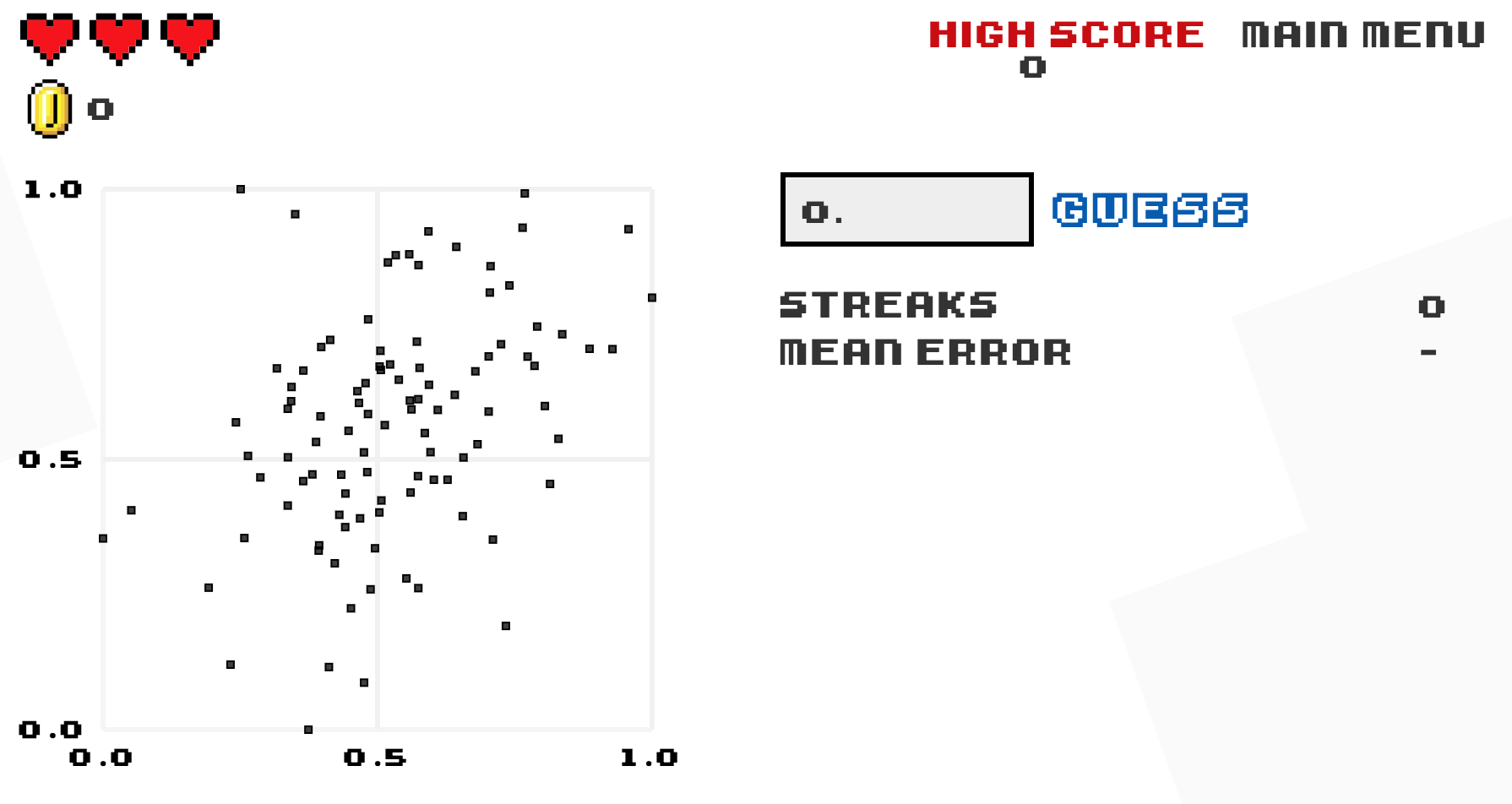FIGURE 5.14: Preview of “Guess the Correlation” game.

### 5.4.2 What’s to come?

In this chapter, you’ve studied the term basic regression, where you fit models that only have one explanatory variable. In Chapter 6, we’ll study multiple regression, where our regression models can now have more than one explanatory variable! In particular, we’ll consider two scenarios: regression models with one numerical and one categorical explanatory variable and regression models with two numerical explanatory variables. This will allow you to construct more sophisticated and more powerful models, all in the hopes of better explaining your outcome variable $$y$$.

### References

Firke, Sam. 2023. Janitor: Simple Tools for Examining and Cleaning Dirty Data.
Grolemund, Garrett, and Hadley Wickham. 2017. R for Data Science. First. Sebastopol, CA: O’Reilly Media. https://r4ds.had.co.nz/.
James, Gareth, Daniela Witten, Trevor Hastie, and Robert Tibshirani. 2017. An Introduction to Statistical Learning: With Applications in r. First. New York, NY: Springer.
Quinn, Michael, Amelia McNamara, Eduardo Arino de la Rubia, Hao Zhu, and Shannon Ellis. 2019. Skimr: Compact and Flexible Summaries of Data. https://github.com/ropenscilabs/skimr.
Robinson, David, Alex Hayes, and Simon Couch. 2023. Broom: Convert Statistical Objects into Tidy Tibbles.
———. 2023. Tidyverse: Easily Install and Load the Tidyverse.